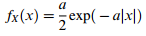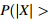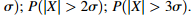Create an Account

Home / Questions / Speech is sometimes idealized as having a Laplacian-amplitude pdf That is the amplitude is...

Speech is sometimes idealized as having a Laplacian-amplitude pdf That is the amplitude is distributed according to a Express the variance of X σ2 in terms of a Show your derivation don’t

Speech is sometimes idealized as having a Laplacian-amplitude pdf. That is, the amplitude is distributed according toa. Express the variance of X, σ2, in terms of a. Show your derivation; don’t just simply copy the result given in Table 5.4

b. Compute the following probabilities:Jul 31 2020 View more View LessSubscribe To Get Solution